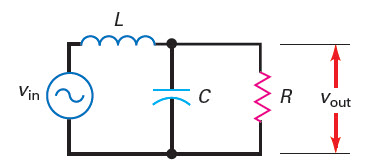# Second Order LC Low Pass Filter Calculator

The following is online calculator for second order LC low pass filter. It calculates cutoff frequency and Q from given inductor, capacitor and load resistor values or calculate inductor, capacitor values from given cutoff frequency.

Resonant Frequency(fr) Calculator
Inputs:

Formula Used:
$$f_{r}=\frac{1}{2 \pi \sqrt{L C}} , Q=\frac{R}{X_L}$$

Theoretical Results:Calculate L or C from fr
Calculate L:
Inputs:

Formula Used:
$$L =\frac{1}{4 \pi^2 f_{r}^2 C}$$

Theoretical Results:

Calculate C:
Inputs:

Formula Used:
$$C =\frac{1}{4 \pi^2 f_{r}^2 L}$$

Theoretical Results:

### Notes on Second Order LC Low Pass Filter Calculator

• Effect of Q
The Q of the filter is given by,

$Q=\frac{R}{X_L}$

where, the inductor reactance $$X_L$$ is calculated at the resonant frequency.

When Q is high the gain will increase and in frequency response graph we will see peak. The following graph illustrates frequency response of LPF when Q is high.

• Higher order LC LPF

Higher order LC low pass filters can be designed by cascading the above 2nd order LPF.

Low pass filter(LPF) is a electrical system that allows low frequency signal to pass through it and blocks the high frequency signal. The above LC low pass filter is a passive filter which means there is no gain of the signal magnitude when the signal is passed through it. This is opposite to active filters which uses operational amplifiers and hence provides gain to signal magnitude at the output of the filter. One of the disadvantage of Passive filter such as this LC low pass filter is that there is loss in signal magnitude when they pass through it. But the advantage of this passive filter is that it can be used at higher frequencies upto giga Hertz where the op-amp based active filters can be used.